Learning Library

5th Grade Fraction Practice

Fraction math can be hard to get a handle on, but with a little practice even the toughest problems can be solved. Help your fifth grader master fractions with these easy-to-follow worksheets.

Click on a worksheet in the set below to see more info or download the PDF.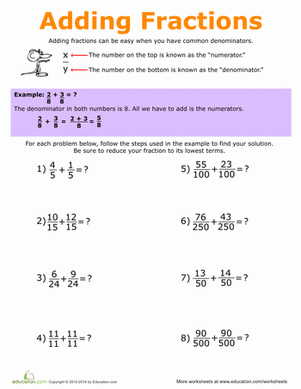How to Add Fractions

A helpful guide and practice questions for adding fractions with like and unlike denominators.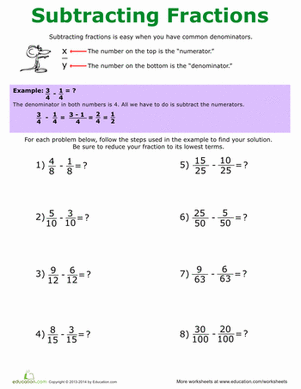How to Subtract Fractions

This worksheet has a step-by-step guide to subtracting fractions with common and uncommon denominators.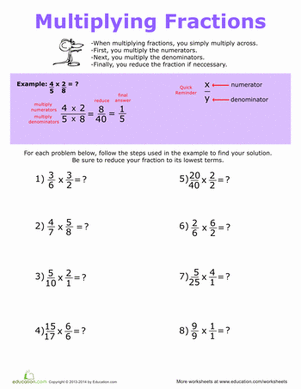How to Multiply Fractions

Use this helpful guide to learn and practice multiplying fractions.Fraction Review: Addition, Subtraction, and Inequalities

Covering everything from adding and subtracting fractions to fraction inequalities, this worksheet offers a great review.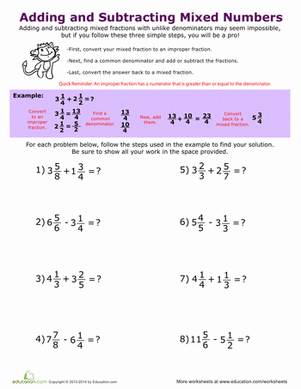Adding and Subtracting Mixed Numbers

Adding and subtracting mixed numbers can be daunting, but this worksheet helps by breaking the process down step by step.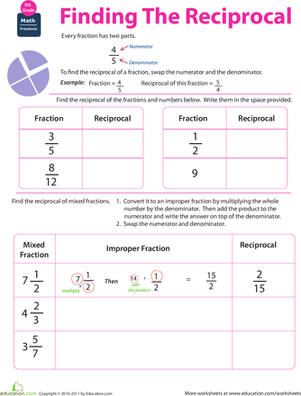Fraction Math: Find the Reciprocal

Help your child wrap his head around this math concept by helping him practice finding the reciprocal of simple fractions and mixed fractions.Improper Fractions

Learn all about improper fractions and how to convert them into mixed numbers.Mixed and Improper Fractions

Learn how to convert mixed fractions to improper fractions in this worksheet.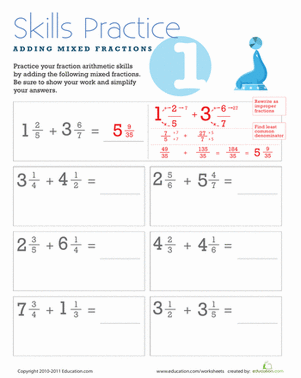This colorful math worksheet will jog your child's memory through the steps to complete mixed fraction addition!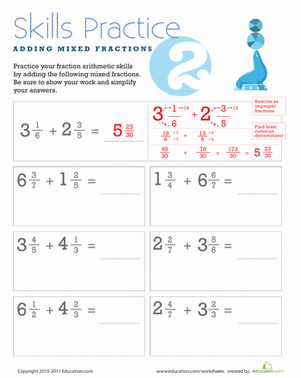Adding Mixed Fractions with Unlike Denominators

Does your child need extra math practice? This worksheet can help her with the steps to adding mixed fractions with unlike denominators.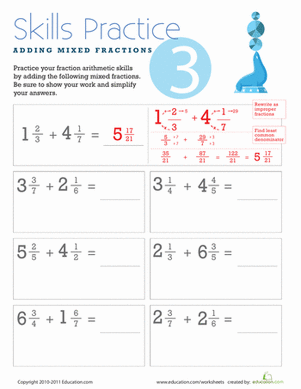Adding Mixed Fractions with Different Denominators

Does your child need extra math practice? This worksheet can help him with the steps to solving mixed fractions addition problems.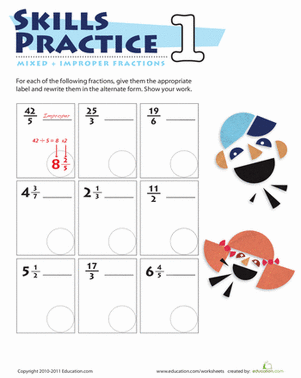Mixed & Improper Fractions 1

This math printable might look silly but that's the point! Keep things interesting for your 5th grader as she practices mixed and improper fractions.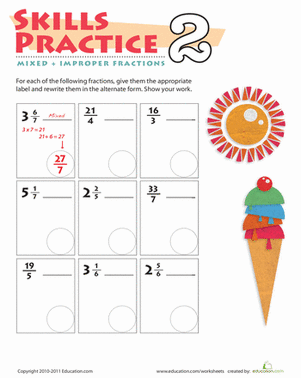Practice Mixed & Improper Fractions 2

This colorful math printable keeps learning interesting for your 5th grader as he practices identifying mixed fractions and improper fractions.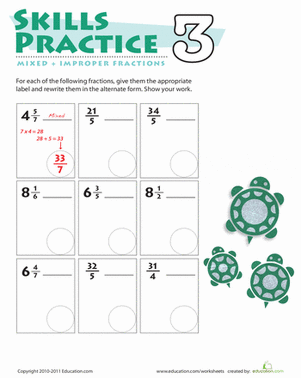Practice Mixed & Improper Fractions 3

This colorful math printable keeps learning fun for your 5th grader as he practices identifying mixed fractions and improper fractions.

Want to download this whole set as a single PDF?

Create new collection

0

New Collection>

0 items

What could we do to improve Education.com?

Please note: Use the Contact Us link at the bottom of our website for account-specific questions or issues.

What would make you love Education.com?